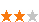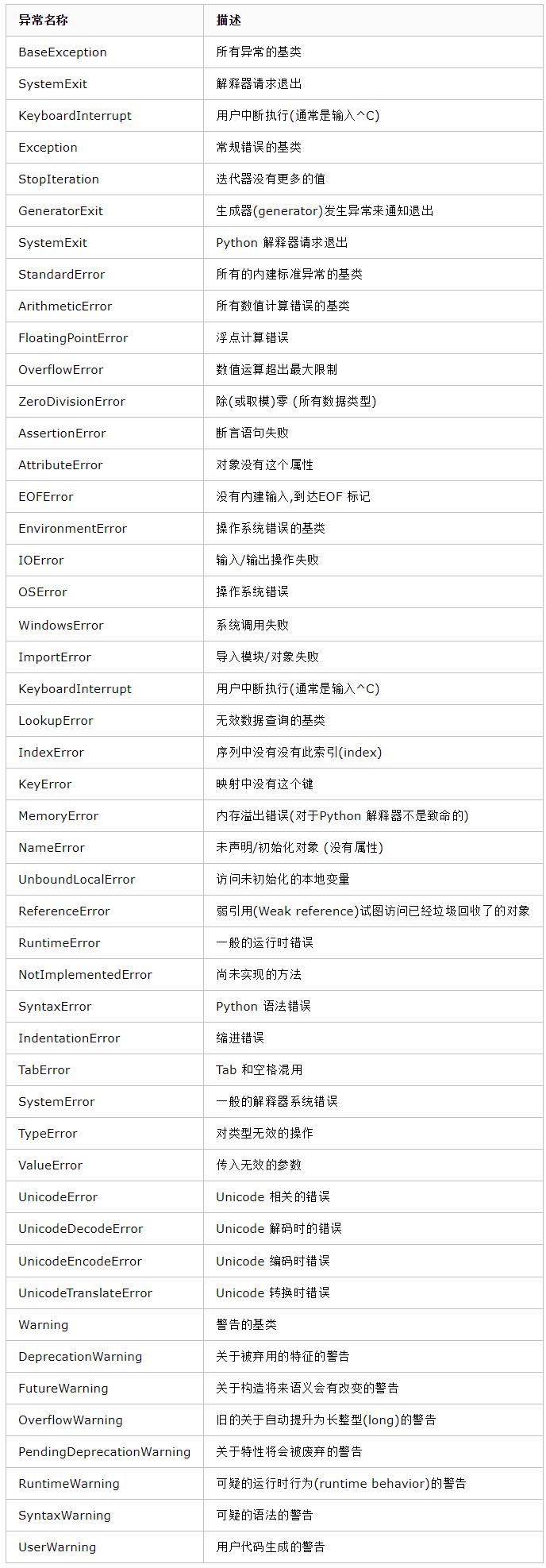您可以捐助，支持我们的公益事业。 1元 10元 50元 认证码：必填求知 文章 文库 Lib 视频 iPerson 课程 认证 咨询 工具 讲座 Modeler Code要资料订阅捐助
python异常处理及内置模块500 次浏览 评价： 好 中 差
2019-9-2

 编辑推荐: 本文来自于cnblogs,文章主要介绍了python异常处理的实例，python标准异常类以及内置模块等，希望对您能有所帮助。

 #!/usr/bin/env python a = 2/0 print(a)

 Traceback (most recent call last): File "002.py", line 2, in a = 2/0 ZeroDivisionError: integer division or modulo by zero

 try: a = 2/0 print(a) except Exception as e: print("除数不能为0") #raise e # 把异常抛出来 finally: print("无论发生什么情况，都执行此步。")

python标准异常类：a.py #!/usr/bin/env python def hello(): print("hello") hello() def world(): print("world") world() b.py #!/usr/bin/env python import a

hello

world

 a.py #!/usr/bin/env python def hello(): print("hello") def world(): print("world") if __name__ == "__main__": hello() world() b.py #!/usr/bin/env python import a a.hello() a.world()

hello

world

1、文件夹里面需要有__init__.py文件的才能当做模块使用。

2、if __name__ == "__main__":语句的使用。

datetime

 import datetime # 下面我们使用的是datetime模块下面的datetime模块，所以使用的时候需要datetime.datetime，为了更方便的使用，也可以直接使用from datetime import datetime print(datetime.datetime.now()) # 打印当前时间 2018-04-23 09:33:32.055974 print(datetime.datetime.now().year) # 打印当前时间中的年份 2018 print(datetime.datetime.now().month) # 打印当前时间中的月份 4 print(datetime.datetime.now().day) # 打印当前时间中的天 23 print(datetime.datetime.now().hour) # 打印当前时间中的小时 9 print(datetime.datetime.now().minute) # 打印当前时间中的分钟 33 print(datetime.datetime.now().second) # 打印当前时间中的秒 32 print(datetime.datetime.now().microsecond) # 打印当前时间中的毫秒 56063 print(datetime.datetime.now().strftime("%Y-%m-%d")) # 从时间格式转换成字符串，满足"%Y-%m-%d"格式的字符串格式 2018-04-23 09:33:32.055974 --> 2018-04-23 print(datetime.datetime.now().strftime("%c")) # 标准时间，类似于这种格式 Mon Apr 23 09:50:45 2018 print(datetime.datetime.now().strftime("%a")) # 本地简化星期名称 Mon print(datetime.datetime.now().strftime("%b")) # 本地简化月份名称 Apr print(datetime.datetime.now().strftime("%d")) # 当前这天是一个月中的第几天 23 # 直接导入datetime模块下面的datetime #from datetime import datetime #print(datetime.now())

%Y 带世纪部分的十进制年份

%m 十进制表示的月份

%d 十进制表示的每月的第几天

%H 24小时制的小时

%M 十进制表示的分钟数

%S 十进制的秒数

 #!/usr/bin/env python from datetime import datetime from datetime import timedelta now_time = datetime.now() # 当前时间 print(now_time) b = now_time + timedelta(days = -1) # 一天前 print(b) c = now_time + timedelta(days = -1,weeks = -1) # 一个周前的前一天 print(c)

2018-04-23 10:35:40.245370

2018-04-22 10:35:40.245370

2018-04-15 10:35:40.245370

time模块

 #!/usr/bin/env python import time time.sleep(2) # 暂停2秒后，打印 print("Hello") print(time.time()) # 打印时间戳，即从1970-01-01到现在的秒数 print(time.localtime()) # time.struct_time(tm_year=2018, tm_mon=4, tm_mday=23, tm_hour=10, tm_min=47, tm_sec=59, tm_wday=0, tm_yday=113, tm_isdst=0) time.strptime(string,[,format]) # 把一个格式化时间字符串转化为struct_time，它和strftime是逆操作。

commands模块

 #!/usr/bin/env python import commands output = commands.getoutput("ls -ll") # 返回执行完命令后的结果 print(output) status, output = commands.getstatusoutput("ls -l") # 返回一个元组，如果shell执行成功，第一个值（状态码）是0表示成功，第二个值是shell执行结果 print(status,output)

subprocess模块

 #!/usr/bin/env python from subprocess import PIPE,Popen p = Popen(['ifconfig'],stdout=PIPE) data = p.stdout.read() print(data)500 次浏览     评价： 好 中 差订阅捐助
 相关文章 手机软件测试用例设计实践 手机客户端UI测试分析 iPhone消息推送机制实现与探讨 Android手机开发（一）
 相关文档 Android_UI官方设计教程 手机开发平台介绍 android拍照及上传功能 Android讲义智能手机开发
 相关课程 Android高级移动应用程序 Android系统开发 Android应用开发 手机软件测试